Function Repository Resource:

# FunctionJectivity

Determine the injectivity and surjectivity of a function

Contributed by: Wolfram|Alpha Math Team
 ResourceFunction["FunctionJectivity"][expr,x,"prop"] determines whether expr, viewed as a function of x, has the "jectivity" property "prop". ResourceFunction["FunctionJectivity"][{expr,cond},x,"prop"] determines whether expr has the given property when x is restricted to satisfy the condition cond.

## Details and Options

For the purposes of determining injectivity and surjectivity, expr is viewed as a mapping from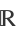to.
The string argument "prop" can take the values "Injective", "Surjective" or "Bijective".
An injective function is also known as "one-to-one" and a surjective function is also known as "onto". A function is called bijective if it is both injective and surjective.

## Examples

### Basic Examples (3)

Test a trigonometric function for injectivity:

 In:=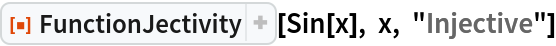Out=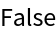Test a rational function for injectivity:

 In:=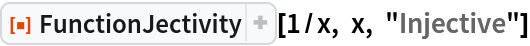Out=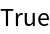Test a polynomial function for surjectivity:

 In:=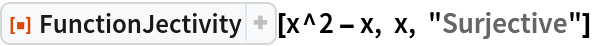Out=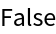### Properties and Relations (4)

Power functions are both injective and surjective for odd powers:

 In:=Out=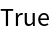Power functions are neither injective nor surjective for even powers:

 In:=Out=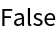In:=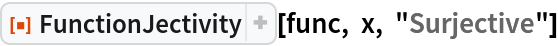Out=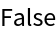A function that is surjective (whose image is the set of reals) may not be so when the domain is restricted:

 In:=Out=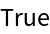Restricting to the domain of positive x, the function is no longer surjective:

 In:=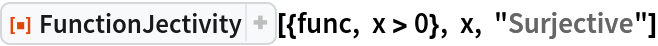Out=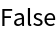A function that is not injective (one-to-one) across the full set of reals may become so when the domain is restricted:

 In:=Out=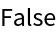Test a power function for injectivity when restricted to the domain of positive x:

 In:=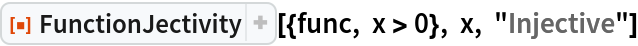Out=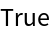## Publisher

Wolfram|Alpha Math Team

## Version History

• 4.0.0 – 23 March 2023
• 3.0.0 – 24 April 2020
• 2.0.0 – 24 January 2020
• 1.0.0 – 17 September 2019

## Author Notes

As a convenience, “prop" can also be among the set {"Injectivity", "Bijectivity", "Surjectivity"}.
It should be extended to give an Association of results when All is used as the property.